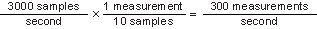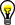# NI PXI-4110 Measurement Averaging

NI DC Power Supplies and SMUs Help (NI-DCPower)

Edition Date: January 2018

Part Number: 370736U-01

View Product InfoNI-DCPower (English | Japanese)

The NI PXI-4110 averages measurement samples to reduce noise and improve sensitivity. You can set the number of samples to average programmatically using the niDCPower Samples To Average property or the NIDCPOWER_ATTR_SAMPLES_TO_AVERAGE attribute.Note  When you set the niDCPower Samples To Average property or the NIDCPOWER_ATTR_SAMPLES_TO_AVERAGE attribute, the output channel measurements may move out of synchronization. Refer to the niDCPower Reset Average Before Measurement property or the NIDCPOWER_ATTR_RESET_AVERAGE_BEFORE_MEASUREMENT attribute for more information about measurement averaging and synchronization.

## Determining Measurement Rate

Although the measurement speed of the NI PXI-4110 is 3 kS/s for all voltage and current measurements, the measurement rate of the NI PXI-4110 can vary depending on the setting of the niDCPower Samples To Average property or the NIDCPOWER_ATTR_SAMPLES_TO_AVERAGE attribute.

The default value of the niDCPower Samples To Average property and the NIDCPOWER_ATTR_SAMPLES_TO_AVERAGE attribute is 10. As expressed in the following equation, the NI PXI-4110 returns 300 measurements per second using the default value:If no measurement averaging is used (Samples To Average = 1), the NI PXI-4110 returns 3,000 measurements per second.

While measuring without averaging yields the fastest measurement rate, noise from the environment (for example, the 50 Hz or 60 Hz noise introduced by cabling) increases measurement uncertainty.

Adjust the niDCPower Samples To Average property or the NIDCPOWER_ATTR_SAMPLES_TO_AVERAGE attribute as necessary to optimize the noise performance and measurement rate for your application.Note  Measurement rate refers only to the hardware measurement rate and does not include software latency.

## Rejecting Noise

If you know the noise frequency, you can reject it from the signal. To determine the number of measurements necessary to reject noise from a signal, divide the measurement speed of the NI PXI-4110 by a full wavelength cycle of noise.

Number of Samples to Average Frequencies Rejected for 3 kHz Sample Rate
1 3 kHz
50 60 Hz
60 50 Hz
300 50 Hz and 60 Hz

For frequencies not listed in this table, use the following formula:

N = 3000/Fr

where N is the number of samples to average and Fr is the frequency rejected.Note   To improve noise reduction while keeping frequency rejection, set the number of samples to average to N, 2N, 3N, and so on. The maximum allowed samples to average is 511.

### Example 1

To reject 60 Hz noise frequency from a signal, average 50 measurements (3 kHz/60 Hz = 50).

### Example 2

To reject 50 Hz noise frequency from a signal, average 60 measurements (3 kHz/50 Hz = 60).Tip  Set the niDCPower Samples To Average property or the NIDCPOWER_ATTR_SAMPLES_TO_AVERAGE attribute to 300, an even multiple of both 60 kHz and 50 kHz, to actively reject both noise frequencies.